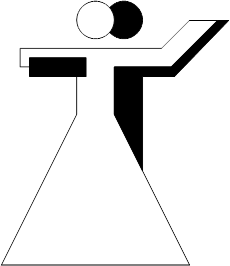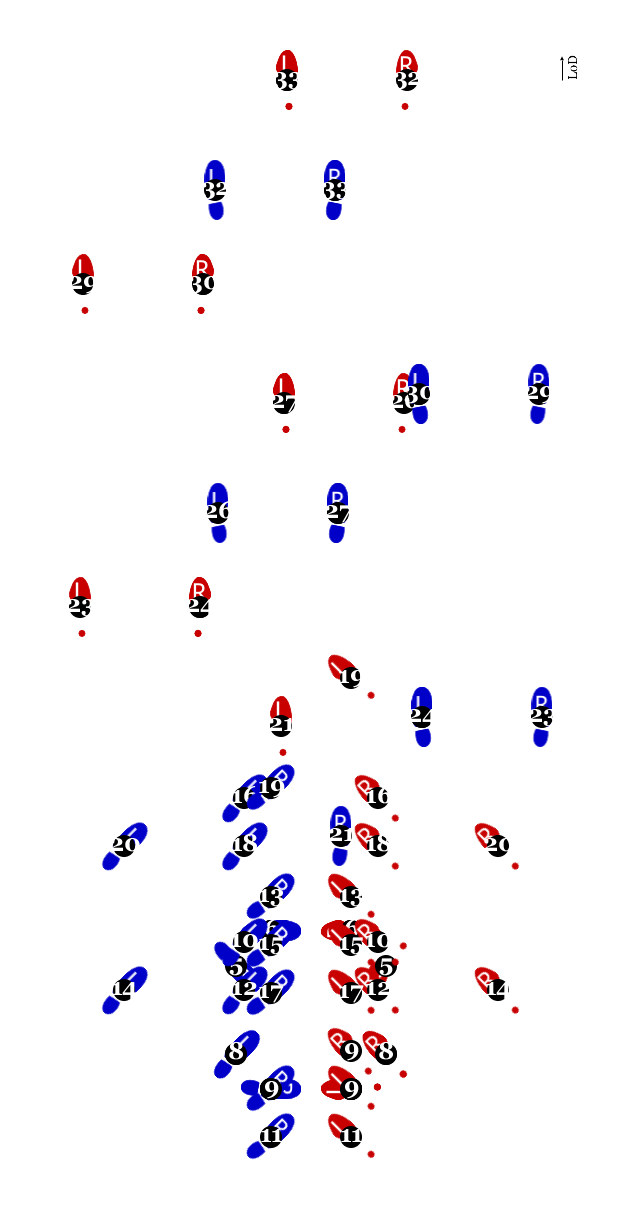# Amalgamation #1en [de]

Sequence with whisk, promenade, and Botafogos## Steps (man)

0 Begin in closed hold, facing the wall, weight on the LF.
1 Take a step to the right (RF). [slow]
2 Cross the LF behind the RF. [a]
3 Put the weight back onto the RF. [slow]
4 Take a step to the left (LF). [slow]
5 Cross the RF behind the LF. [a]
6 Put the weight back onto the LF. [slow]
7 Take a step to the right (RF). [slow]
8 Cross the LF behind the RF. [a]
9 Put the weight back onto the RF and open in promenade position. [slow]
RF in place. Make an eight turn to the left.
10 Take a step diagonally forward and to the left (LF). [slow]
11 Take a small step backward (RF). [a]
12 Slip the LF backward. [slow]
13 Take a step diagonally forward and to the left (RF). [slow]
14 Take a small step to the side (LF). [a]
15 Slip the RF backward. [slow]
16 Take a step diagonally forward and to the left (LF). [slow]
17 Take a small step backward (RF). [a]
18 Slip the LF backward. [slow]
19 Take a step diagonally forward and to the left (RF). [slow]
20 Take a small step to the side (LF). [a]
21 Slip the RF backward. [slow]
Take a step diagonally forward and to the right (RF).
RF in place. Make an eight turn to the left.
22 Take a step diagonally forward and to the right (LF). [slow]
23 Take a step to the right (RF). [a]
24 Put the weight back onto the LF. [slow]
25 Take a step diagonally forward and to the left (RF). [slow]
26 Take a step to the left (LF). [a]
27 Put the weight back onto the RF. [slow]
28 Take a step diagonally forward and to the right (LF). [slow]
29 Take a step to the right (RF). [a]
30 Put the weight back onto the LF. [slow]
31 Take a step diagonally forward and to the left (RF). [slow]
32 Take a step to the left (LF). [a]
33 Put the weight back onto the RF. [slow]

0 Begin in closed hold, facing the centre, weight on the RF.
1 Take a step to the left (LF). [slow]
2 Cross the RF behind the LF. [a]
3 Put the weight back onto the LF. [slow]
4 Take a step to the right (RF). [slow]
5 Cross the LF behind the RF. [a]
6 Put the weight back onto the RF. [slow]
7 Take a step to the left (LF). [slow]
8 Cross the RF behind the LF. [a]
9 Put the weight back onto the LF and open in promenade position. [slow]
RF in place. Make an eight turn to the right.
10 Take a step diagonally forward and to the right (RF). [slow]
11 Take a small step backward (LF). [a]
12 Slip the RF backward. [slow]
13 Take a step diagonally forward and to the right (LF). [slow]
14 Take a small step backward (RF). [a]
15 Slip the LF backward. [slow]
16 Take a step diagonally forward and to the right (RF). [slow]
17 Take a small step backward (LF). [a]
18 Slip the RF backward. [slow]
19 Take a step diagonally forward and to the right (LF). [slow]
20 Take a small step backward (RF). [a]
21 Slip the LF backward. [slow]
Take a step diagonally forward and to the left (LF).
LF in place. Make an eight turn to the right.
22 Take a step diagonally forward and to the left (RF). [slow]
23 Take a step to the left (LF). [a]
24 Put the weight back onto the RF. [slow]
25 Take a step diagonally forward and to the right (LF). [slow]
26 Take a step to the right (RF). [a]
27 Put the weight back onto the LF. [slow]
28 Take a step diagonally forward and to the left (RF). [slow]
29 Take a step to the left (LF). [a]
30 Put the weight back onto the RF. [slow]
31 Take a step diagonally forward and to the right (LF). [slow]
32 Take a step to the right (RF). [a]
33 Put the weight back onto the LF. [slow]

## Following figures

Verbal and animated descriptions of latin and ballroom dancing figures